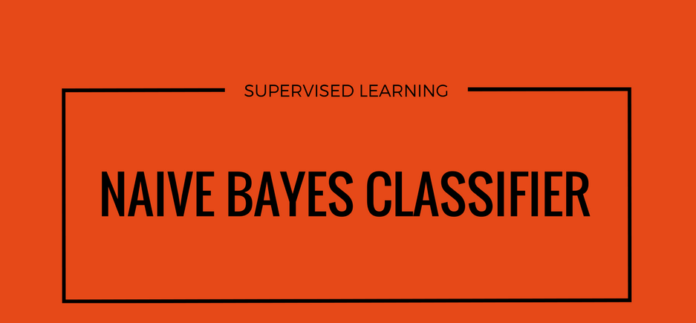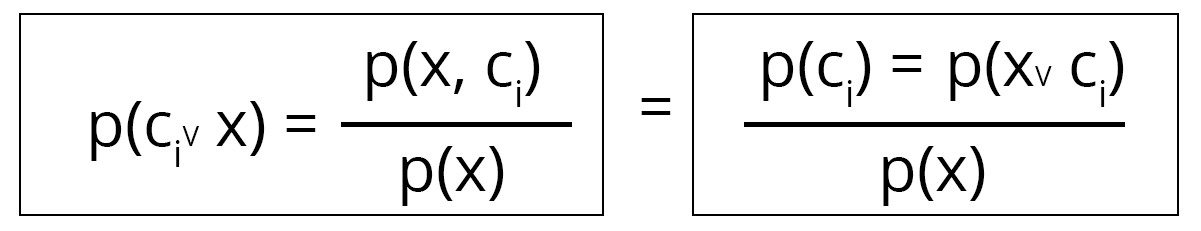# Spam Detection using Naive Bayes Algorithm

1
2746Every day, we receive tons of irrelevant emails, some of which are simply marketing/promotional emails while others are fraudulent ones. The top tech companies like Google, etc spend a lot of manpower in developing robust spam classifiers simply because customers are annoyed with such emails. Here is an example spam email:

Dear Mr. Aman,
You have won a lottery offer for \$2,000,000!!! Click here to claim it now.

Clearly, this is a spam email for most cases. The sender of such email wants you to click on the link so that he/she could fool you by:

Spam detection problem is therefore quite important to solve. More formally, we are given an email or an SMS and we are required to classify it as a spam or a no-spam (often called ham).

Naive Bayes Algorithm

Naive Bayes is a simple Machine Learning algorithm that is useful in certain situations, particularly in problems like spam classification. It is based on the famous Bayes Theorem of Probability.

We are given an input vector X = [x1, x2, x3 .., xn] which could be classified into one of the k classes C1, C2 .. Ck. To achieve this classification, we use the Naive Bayes algorithm to model the probabilities P(Ci | X) for each of the classes C1, C2 .. Ck. Then, we label the class of X as Ci such that P(Ci | X) is maximum. Basically, the class whose probability comes to be highest given the sample X. To calculate this probability P(Ci | X) we use the Bayes theorem:Clearly, P(X) remains a constant for a given value of X. So, we only need to compute the numerator of the middle part: P(X, Ci). This can be written as:

P(X, Ci) = P(x1, x2, x3 .., xn, Ci)
= P(x1 | x2, x3 .., xn, Ci) x P(x2, x3 .., xn, Ci)
= P(x1 | x2, x3 .., xn, Ci) x P(x2 | x3 .., xn, Ci) x P(x3 .., xn, Ci)
..
..

If we assume that each feature xi is conditionally independent of the other features, we can simplify the above as:

= P(x1) x P(x2) .. x P(xn) x P(Ci)

So, essentially we have P(Ci | X) = (1/K) P(Ci) x [P(x1) x P(x2) .. x P(xn)], where K = P(X)

The probability P(Ci) can easily be estimated from the training data by measuring the number of sample points in each of the classes Ci. Similarly, the probabilities P(x1), P(x2) .. P(xn) etc can also be estimated from the dataset by looking at the number of sample points where they occur respectively.

The name Naive Bayes comes from the assumption above that “each feature xi is conditionally independent of the other features”. In reality, this may not be true. However, for most practical purposes, it holds good.

Naive Bayes applied to Spam Detection problem

In the spam detection problem, there are 2 classes: C1 which is the no-spam (ham) class and C2 which is the spam class. X is essentially each email present in the training data. To convert X into a machine-readable form (number), we basically need to convert X into a vector. We achieve it by the following way:

• Create an ordered list of all the words in the vocabulary. For instance, suppose we have the following words in the vocabulary: [lottery, how, won, offer, thanks, the, you].
• To convert an email into a vector, map out the number of times each word occurs in that email. For instance, consider the following email: you won the lottery. The vector form of the above email would be [1, 0, 1, 0, 0, 1, 1].

Now that we have mapped each email into a vector, we can apply the Naive Bayes algorithm on the data. Observe that in the above process, we assumed that each word is produced independent of each other and we discarded the ordering of words in the email. This exactly is the “Naive” assumption and that’s how we plan to apply the Naive Bayes algorithm to this problem.

Python provides sklearn library which is a powerful Machine Learning library that will help us abstract out the complicated details of the estimation of Naive Bayes probabilities and will rather help us focus on training the algorithm and obtaining the outputs fast.

Download the Kaggle SMS dataset from here and take a look at the code below:

```from sklearn import datasets
from sklearn.naive_bayes import GaussianNB
from sklearn.cross_validation import train_test_split
import pandas as pd

VOCABULARY = set()

# Extract the list of words from an email
def get_words_in_email(email):
words = []
for word in email.split():
if word != '':
word = word.lower().strip()
word = ''.join(ch for ch in word if ch.isalpha())
words.append(word)
return words

# Populate vocabulary by combining all words in the training data
def create_vocabulary(data):
global VOCABULARY
for email in data['v2']:
words = get_words_in_email(email)
for word in words:
VOCABULARY = list(VOCABULARY)

create_vocabulary(data)

# Convert email to vector as explained above
def convert_email_to_vector(email):
words = get_words_in_email(email)
vector_email =  * len(VOCABULARY)
for word in words:
vector_email[VOCABULARY.index(word)] += 1
return vector_email

# Convert all emails to vector
def get_preprocessed_training_data(data):
X = []
Y = []
for i in range(len(data['v2'])):
email = data['v2'][i]
label = data['v1'][i]
X.append(convert_email_to_vector(email))
Y.append(0 if label == 'ham' else 1)
return X, Y

X, Y = get_preprocessed_training_data(data)

# Split the data into training and testing data
X_train, X_test, Y_train, Y_test = train_test_split(X, Y,
test_size=0.3,
random_state=42)

gnb = GaussianNB()

# Get the predictions
predictions = gnb.fit(X_train, Y_train).predict(X_test)
number_of_spam_emails = sum(Y_test)
number_of_non_spam_emails = len(Y_test) - number_of_spam_emails
spam_emails_identified_among_spam = 0
non_spam_emails_identified_among_spam = 0

for i in range(len(predictions)):
if Y_test[i] == 1:
if predictions[i] == 1:
spam_emails_identified_among_spam += 1
else:
non_spam_emails_identified_among_spam += 1

print number_of_spam_emails, number_of_non_spam_emails
print spam_emails_identified_among_spam, non_spam_emails_identified_among_spam
```

As can be seen, the output of the code would be:
219 1453
196 23

So, in the training data, there were 219 spam emails out of which the algorithm was able to correctly identify 196 as spam. This is a staggering 89.5% accuracy which is fantastic for such a simple code.

Hope this blog helped you understand the basics of Naive Bayes and the spam classification problem and that you would be able to implement your own spam classifier.

#### 1 COMMENT

1.sarfaraj mullick

how to check with my own email?